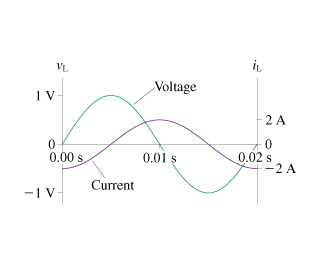# Problem: The figure shows voltage and current graphs for an inductor.What is the emf frequency f?

###### FREE Expert Solution
88% (479 ratings)###### Problem Details

The figure shows voltage and current graphs for an inductor.

What is the emf frequency f?Frequently Asked Questions

What scientific concept do you need to know in order to solve this problem?

Our tutors have indicated that to solve this problem you will need to apply the Impedance in AC Circuits concept. You can view video lessons to learn Impedance in AC Circuits. Or if you need more Impedance in AC Circuits practice, you can also practice Impedance in AC Circuits practice problems.